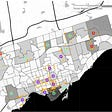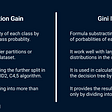# What is support vector machine (SVM) ?

## An introduction to machine learning algorithms

A support vector machine is a popular machine learning model today in this article, I would be giving you a detailed explanation and how this model works.

support vector model comes in the field of supervised learning.

# Support vector machine and regression

Support vector model can be used for both problems regression as well as classification and it’s divided into 2 parts support vector machine (SVM) is used for classification problems and support vector regression (SVR) is mostly used for regression problems but in this article, I would be telling you about support vector machine (SVM) probably in the next article I would be talking about support vector regression (SVR)
so first of all

# How does it work

SVM finds a hyperplane in an n-dimension or a line to classify between the dataset so when we add a new point in the dataset the SVM can tell what class the point belongs to

so let’s say we have a dataset of multiple points and belong to 2 types of classes so how does SVM separate the classes from each other ?

it creates 2 lines or hyperplanes in the nth dimension with maximum margin one will be positive and the other will be negative in the given representation you can see how this model works

## What is a hyperplane?

the hyperplane is the boundary between two classes that separates them from each other and the hyperplane can be dependent on the dataset if our data is 2 dimensional the hyperplane will be a line and if our data is 3 dimensional then the hyperplane will be a 2d plane which separates the data points

## What are support vectors?

support vectors are the closest point that will decide the position of a hyperplane the point touching the positive and negative hyperplane and called support vectors because they are the foundation of the hyperplane

# Types of SVM

## linear SVM

linear SVM when our data is linear then linear SVM and we can separate them from a straight line

## non-linear SVM

non-linear SVM when our data is non-linear then we use non-linear SVM and it is not separable by a straight line but instead can be separated by a curve

# example

## linear SVM

linear SVM when our data something looks like this or we can separate them by a straight line

then there are many lines we can draw that can separate our data but to find the best line

we need to introduce a hyperplane which consists of mostly all the lines which separates the data and support vectors helps them to build the hyperplane

## non-linear SVM

non-linear SVM in the linear SVM we used a line to separate our data but in non-linear SVM when our data looks something like this and cannot be separated by a line we need to introduce a curve to separate the data

so to separate these points we will be adding one more dimension to our data we have used x and y dimensions now we will introduce a z dimension to where z points will be decided by the formula
z = x² +y²

now our transformed data would look something like this and now we can separate our dataset by a hyperplane

it’s in 3D so you see it as a line but when we convert it into 2D we will get that our data is separated by a circle

## conclusion

so I hope today you guys have a good understanding of support vector machine in the near future I would be making more articles in which I will be explaining more models.

--

--

--

## More from Aviral Bhardwaj

writer

Love podcasts or audiobooks? Learn on the go with our new app.

## Wearables in Review: Polar Vantage V2## Machine Learning on the Road to Predictive Analytics## Housing Prices Analysis in California’s Desert Cities.## Found This Week #68## ML 101: Understanding ROC curves## Building a Data Science Organization## How to: Run a historical basket calculation (TUTORIAL) | Index One## The Battle of the Neighborhoods of Toronto: Restaurantswriter

## What is Supervised Learning?## Gradient Descent: 🗻Going downhill Pt 1## All About Decision Trees In Data Mining## KNN- Machine Learning Algorithm.